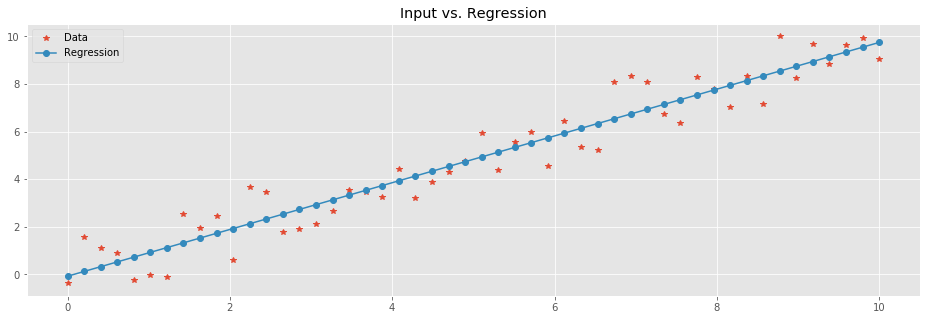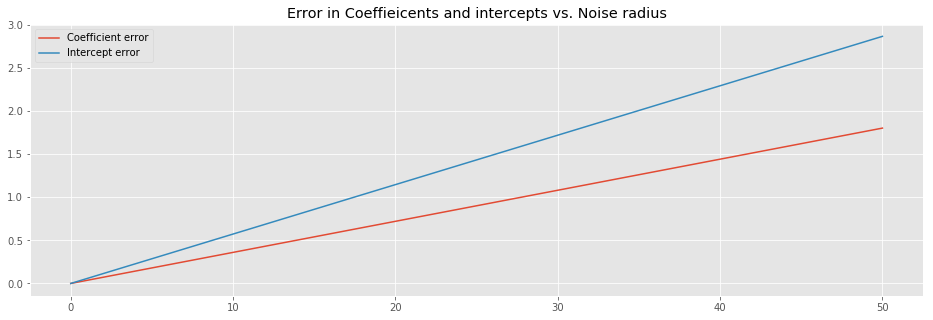# Dr. Dror

Foo is not just a "Bar"

# Minimal example with linear regression

(Original notebook can be found in this gist)

import numpy as np
from sklearn import linear_model
import matplotlib.pyplot as plt
%matplotlib inline
plt.rcParams['figure.figsize'] = (16.0, 5.0)
plt.style.use('ggplot')


This is a rather straightforward example of how to train a linear regression model in a 2D case. $$X$$ is a vector with the independent variables and $$Y$$ with the dependent ones. In this particular example we synthetically generate points on a linear function while adding noise.

X = np.linspace(0,10,50)
X[:3] # sample of X

array([ 0.        ,  0.20408163,  0.40816327])

np.random.seed(42)
noise = 1.5*(2*np.random.random(len(X)) - 1)
Y = (X + noise)
Y[:3] # sample of Y

array([-0.37637964,  1.55622455,  1.10414509])


We start an instance of a linear regression model.

regr = linear_model.LinearRegression()


We train it on the synthetic data.

regr.fit(X.reshape(-1,1),Y)

LinearRegression(copy_X=True, fit_intercept=True, n_jobs=1, normalize=False)


The reshape above is there due to a warning/error you might be seeing if omitted.

Once trained, the model can be used to predict the values of the points we started with in $$X$$. Note, as we are dealing with a linear model, there is no way of ending up with over-fitting model.

Y_pred = regr.predict(X.reshape(-1,1))

plt.plot(
X,Y,'*', # Data
X, Y_pred,'-o' # Regression
)
plt.legend(['Data','Regression'])
plt.title('Input vs. Regression');Let's look into the result. The linear function to which we added noise was $$y=ax + b$$ where the coefficient $$a = 1$$ and the intercept $$b=0$$. We can compute $$a$$ and $$b$$ of the trained model. $$a$$, a.k.a. the slope, is directly computed by:

(Y_pred - Y_pred)/(X - X)

0.98216273101406792


It is actually an attribute of the model:

regr.coef_

array([ 0.98216273])


Similarly, the intercept is merely the value of the model for $$X=0$$:

Y_pred

-0.073041941893555951


And again, this is an attribute of the model:

regr.intercept_

-0.073041941893555951


In the example so far, the noise was uniformly distributed in the $$[-1.5,1.5)$$ interval. Next, we'd like to check the relation between the radius ($$1.5$$ in this case) of the noise and the coefficient/intercept of the model.

def gen_data(size=30, noise_radius=1.5, seed=42):
X = np.linspace(0,10,size)
np.random.seed(seed)
Y = (X + noise)
return X, Y

def train_predict(X, Y):
regr = linear_model.LinearRegression()
regr.fit(X.reshape(-1,1),Y)
Y_pred = regr.predict(X.reshape(-1,1))
return regr, Y_pred


Next we are going to generate synthetic data sets with growing noise radius. In each iterations, we will register the coefficient and the intercept of the trained model.

coeff = []
inter = []
regr, Y_pred = train_predict(X,Y)
coeff.append(regr.coef_)
inter.append(regr.intercept_)


Let's have a look at the behavior of the error between the coefficients and intercepts that we witness and the real ones we used for the generation of the data.

plt.plot(
)
plt.title('Error in Coeffieicents and intercepts vs. Noise radius')
plt.legend(['Coefficient error', 'Intercept error']);The above image suggest that the error of both the coefficient and the intercept linearly depend on the noise radius.

Formally, the we have samples from a linear function

$$y = x + \epsilon$$

where $$\epsilon$$ is uniform noise. For each $$x_i \in X$$ we have a corresponding value $$y_i \in Y$$. Our objective is to find $$a$$ and $$b$$ such that $$y \sim ax +b$$. We do so by minimizing the $$L_2$$ error

$$E(a,b) = \sum_{i=1}^n (y_i - (ax_i+b))^2$$

as a function of $$a$$ and $$b$$. We take partial derivatives and find the minimum:

$$\left\{ \begin{array}{c} \frac{\partial E}{\partial a} = 0 \\ \frac{\partial E}{\partial b} = 0 \end{array} \right.$$

This turns to be:

$$\left\{ \begin{array}{c} \sum (y_i - (ax_i +b))x_i = 0 \\ \sum (y_i - (ax_i +b)) = 0 \end{array} \right.$$

From this, we can have a closed formula for $$a$$ and $$b$$:

$$\left\{ \begin{array}{c} a = \frac{X \cdot Y - n \bar{X}\bar{Y}}{X \cdot X - n \bar{X}^2} \\ b = \bar{Y} - a \bar{X} \end{array} \right.$$

Here, $$\bar{X}$$ and $$\bar{Y}$$ denote the mean of $$X$$ and $$Y$$, respectively. Furthermore, $$n$$ is the number of sample points. We can now check the closed formulas, and compare them to the coefficient and intercept of the model we tarined:

(np.dot(X,Y)/(len(Y)) - X.mean()*Y.mean())/\
(np.dot(X,X)/len(X) - np.power(X.mean(),2))

-0.8010271422548586


vs.

regr.coef_

array([-0.80102714])


And:

Y.mean() - X.mean()* \
(np.dot(X,Y)/(len(Y)) - X.mean()*Y.mean())/\
(np.dot(X,X)/len(X) - np.power(X.mean(),2))

2.8648629210179717


vs.

regr.intercept_

2.864862921017969


Lastly, the closed formulas above provide good intuition why the error of the model grows linearly. In the future I will explain this in details.